## Regulators in Use & Misuseby Harry Lythall - SM0VPO## Introduction

Today one can buy simple voltage regulators for just \$1 and they are perfect for a quick solution for regulating voltages to sensitive circuits, such as oscillators and logic circuits. TTL and ECL integrated circuits that, for example, need to have a regulated 5 Volt supply, within +/-0.25 Volts. LiPo batteries can be burnt if the charging voltage exceeds 4.1 Volts, and free-running oscillators have a tendency to drift if the supply voltage is allowed to vary.

Voltage regulators are so cheap and simple to use that they can be put to use in other circuits, or extended to give better performance. They can also be "misused", or put to use in other applications for which they were never intended. In this article I will attempt to give you an introduction to their use and misuse. If you should find another unusual use for them then please let me know and I will extend this article. Mail me: Harry Lythall harry(.)lythall(at)sm0vpo(.)com.

These regulator Integrated Circuits (IC's) come in different "flavours". The last two digits specify the output voltage. In this article, with one exception, I will use the 78L05, which is a 5V regulator.

DeviceOutput voltageOutput current
78L02 2 Volts100mA
78L05 5 Volts100mA
78L06 6 Volts100mA
78L08 8 Volts100mA
78L09 9 Volts100mA
78L1010 Volts100mA
78L1212 Volts100mA
78L1515 Volts100mA
LM317HPVariable (1.2v)500mA

These devices can cost you typically \$1 each, but if you can use just one value then you can get 50 pieces for \$25. I bought mine in batches of 1000 so if you need them quickly and cheaply then I can let you have small quantities at 5 pieces for 1\$ plus \$2 postage (any quantity). Mail me if you want any. Sorry but I cannot let you have more than 50 pieces.## Basic Information

The first and most obvious use for a voltage regulator chip is to regulate voltage. I have a huge stock of 78L05 and 78L10 chips that I bought from China. These devices have three wires: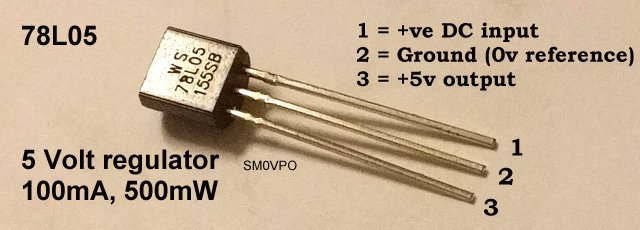Outline of the 78L05 and LM317 regulators

Pin numberfunction
1Input voltage
2Ground/Common (0 Volts reference)
3Output voltage

The 0 Volt reference (common) pin-2 draws almost no current, it is just a voltage senor pin. In effect the input current is equal to the output current. Inside the chip there is quite a lot of electronics. Not exactly LSI, but you do get a lot for your money.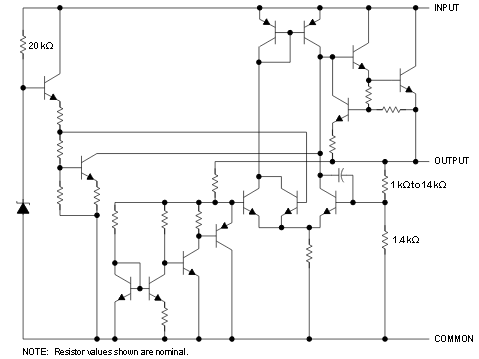Internal circuit of the 78L05 regulator

POWER RATING
The chips I use look like a simple plastic package transistor, but they contain quite a bit more. They are also rated at maximum 100mA. If you exceed the 100mA current capability then they will shut down. They should also be restricted to a disipation of about 0.5 Watts. If you are regulating 10v down to 5V then the device has a voltage drop of 5V. At 100mA the dissipation will be 5 x 0.1 = 500mW. If you were to regulate a car battery (14 Volts) down to 5V then the device voltage drop will be 14 - 5 = 9 Volts. This means you cannot draw more than about 55mA or the device will get hot.## Basic Voltage Regulator

As a basic positive voltage regulator, you simply connect pin-2 to ground, supply voltage to pin-1 and take your output from pin-3. It couldn't be easier.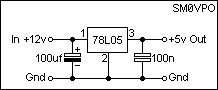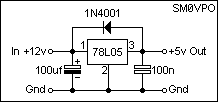Simple voltage regulator - and a - protected regulator

The problem with this over-simplified arrangement is that the equipment supplied requires a low impedance, especially if digital or RF circuits are to be powered where there could be switching transients. To give the output a low AC (AF or RF) impedance you need to put some form of capacitance across the output. The unwanted effect of this is that on initial powering up of the regulator, the capacitor will draw a large current. This is not a problem as the device will restrict this current to 100mA. However, when the power is switched OFF then the capacitor will discharge backwards through the regulator. In extreme cases this can cause a device failure. The solution to this is to add a protection diode between the input and the output. In normal use this diode is reverse biased so it has no effect. But when the input power becomes zero then the diode will conduct the voltage on the capacitor back to the input circuitry, effectively bypassing the discharge current and protecting the device.## High Current Voltage Regulator

There are higher current regulators available, but you can extend the device current capability to many amperes by using a bypass transistor. In this configuration the regulator will function exactly as normal, but when the output current increases, the input current to the device is used to turn on a PNP power transistor, such as the TIP32, TPI42 or 2N2955. The power transistor will then feed the output with most of the required current.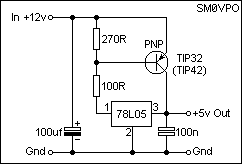Bypassed regulator for high currents

If you need to have more than one output voltage then there is absolutely no reason why you cannot buy several reguators of the same type and cascode connect them. In this application I needed both +5v and +10v so I used two 5v regulators, but the output of one regulator is the 0v reference for the second regulator. The result is a dual-voltage power supply unit, and there is absolutely no reason why either, or both of these two regulators, cannot be bypassed by a power transistor to get high current regulators. Just use the resistor/transistor arrangement as shown in the preveious example.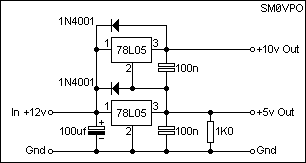One regulator output is the reference for another regulator

Note that there is a 1K0 resistor load on the lower 5v regulator. This is to prevent the small control current from the upper regulator raising the +5V output above 5v. It only takes 5mA, which is a small part of the 100mA output budget. The reference pin (pin-2) current is about 3mA.## Programable Voltage Regulator

The output voltage of the regulator chip is only related to the control connection; pin-2. There is absolutely no reason why you cannot increase the control pin voltage to a potential above ground. This will raise the output voltage of the regulator. You can do this by using a simple zener diode. If you use a zener diode then you may have to add a little current to the zener to get the rated voltage. This you can take from the the chip pin-3 since the voltage is stabilised and therefore the resistor current is constant,and added to the 3mA control pin current. But if you are not worried about 0.1v then you can usually omit this resistor.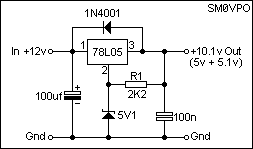Using a zener to change the reguator voltage

Since the current is constant then replacing the zener diode with a resistor will give a voltage offset directly proportional to the resistance. R2 can be made variable, using a linear taper potentiometer. Using this method you can program any voltage you want from 5v upwards.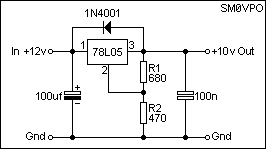Resistive divider to set the output voltage

If you are interedted in the calculations, the 680 Ohm resistor from the 5v regulator output will draw 7.3mA. Add to this the control pin current, 3.2mA, and you get 10.5mA through the 470 Ohm resistor, which will lift the control voltage by 4.95 volts. If you want this to be EXACT then you can add a resistor in series with the 470 Ohm. Every 1 Ohm will give you 10mV (0.01v).In the previous example we used a resistive voltage divider to program the output voltage. R1 will have a constant voltage, which will cause a constant current to flow through R2, plus the control pin current. But you can change R2 for a variable resistor and have a variable voltage regulator. The output voltage varies in direct proportion to the value of R2, so you do not need LOG or Square-law potentiometers; just a bog-standard 470 Ohm linear potentiometer.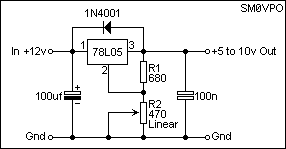Variable resistor to adjust the output voltage## Variable Voltage Regulator

In all my examples I have used a 78L05 5V regulator, so in this example the output voltage can ve varied between +5V and a volt or so under the supply voltage. This may be adequate for a preset Power Supply Unit (PSU), or one that can be adjusted a little. If, however, you want a general purpose PSU for bench use then you may want it to vary from 0V to, say, 10V. Such a PSU will normally have a transformer and diode rectifier. The addition of one low-current signal diode, one resistor and a capacitor can be used to create a -12V supply that can be used to give the regulator chip a -5V reference. The output can then be varied from 0V to 10V.

One small anoying problem I have encountered is that of a variable voltage regulator not being able to deliver Zero Volts. Winding the voltage all the way to zero is in my mind an important feature, but not to worry. A 5.1V zener diode in the -Ve voltage reference will give you 0.1V below zero, which will allow you to have a true 0V - 10V PSU, without any residual voltage at 0V.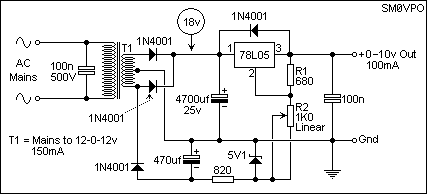Increasing the variable range from 0v to 10v

The 820 Ohm resistor passes about 8mA through the zener diode and another 10mA through the voltage control resistors. You can adjust this resistor to get the zero-volts exact. The 820 Ohm resistor should not be more than about 1.5K Ohms.## Battery Charger

There are two ways you can charge a battery:

• Constant Current - Used for De-Ac, Ni-CAD and LiPO cells.
• Constant Voltage - Used for long-term charging.

Constant current charging is used when you have a cell, or a battery of cells, that need to have a calibrated charge. For example, an AAA cell of 600mA/h capacity would be best be charged at the 10-hour rate (60mA) for 11 hours. If you used a constant voltage or a resitive feed, then as the cell charges, the terminal voltage rises, and the charging current gradually falls. A constant current charger will maintain a calibrated charging current during the entire charge cycle. Cells should be discharged before giving a full charge.

This circuit will generate a constant current. Note that the current will include 3mA extra current from the 78L05 control pin. R1 (in Ohms = 5 divided by the required current (Amperes).Constant current battery cell charger

Constant Voltage, or "float-charging" would be used for maintaining a charge for an indefinate period of time, for example, battery backup in an alarm system, or emergency lighting. The battery will take as much current as it wants (up to the current limit), but when the battery terminal voltage rises, the charging current will decrease to almost nothing. The battery will not be burned by overcharging. A 12 Volt VRLA or lead-acid battery, for example, could be charged 24/7 if the charger is limited to 13.8 V.## High Gain Amplifier

Yes, you can use the voltage regulator with a single transistor to give you a really huge gain in an amplifier. Unfortunately the 3mA quiescent current flowing in the control pin of the 78L05 is not a constant current, so the 78L05 is less suitable in this application. The LM317 is a better alternative where the quiescent current is but a few micro-amperes. But the 78L05 can give a respectful gain.

A single transistor amplifier is a current operated device. In a single transistor amplifier the collector current is only dependant on the base current (Ic = Ib * hfe). The collector voltage has virtually no effect on the current. With the BC547, for example (hfe = about 200), varying the base current by 5uA will cause a (5uA x 200) 1mA change in the collector current. If the collector load resistor were 100 Ohms, then this would give a voltage (signal) change of 0.1 volts. If the collector resistor were raised 10x to 1K Ohms, then the collector voltage would vary by 1 volt.

This means that only resistor value in the collector limits the gain. If you used a 1M0 resistor (1,000,000 Ohms) then the same 1mA variation would give you a (1mA x 1,000,000) 1000V-AC output. This is not practical because you would need to have more than 1000V-DC supply voltage, and not many transistors can stand this voltage. Another point is that as the collector resistor is increased in value, then the output impedance rises, which in turn limits the upper frequency. High voltage and resistance technique was used in the days of thermionic valves (tubes) but it was quite rare to have domestic amplifiers with more than 250 Volts to the anode.

If the collector resistor were replaced by a 1mA constant current source, then it can look like an infinite resistor, but with a practical operating voltage. If the transistor were to draw 1.1mA then the collector voltage would be 0v DC since the constant current source will not deliver more than 1mA. But if the transistor were to draw 0.9mA then the collector voltage would be equal to the supply voltage because it would keep raising the voltage until the current became 1mA, which it will never do. Theoretically the voltage gain of the transistor amplifier would become infinity.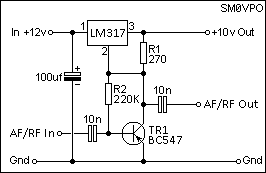LM317 Regulator used as an amplifier

Using this technique the output impedance of the amplifier will still remain relatively low, enabling you to realise an amplifier well into the tens of MHz region. In the above circuit the resistor from the collector to the base will bias the transistor into the linear region where the transistor draws about 5mA. It will also add AC negative feedback, but using a low-impedance input this feedback will be nothing. You could alternatively use two series-connected 100K resistors and have the junction grounded through a capacitor.

With this sort of technique you should be able to realize an AC voltage gain of 100,000, depending on how good the constant current source is.## AF/RF Low-Power Amplifier

If the regulator is used as standard, but the ground sensor terminal (pin-2) is grounded by a transformer, then a signal can be injected to the transformer and the DC output modulated with the AC signal. This AC signal can be audio or radio signals. just use a suitable transformer and it will work. It will work well into the HF spectrum. Mine works at 14 MHz.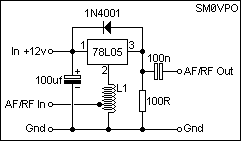Af or RF power amplifier

Using a 78L05 regulator you can only get 10v peak-to-peak, in a class-A linear amplifier. At 50-Ohms this represents about 180mW, which is still a modest QRP signal for the HF bands. Once again you can use a bypass transistor to get a higher current and use an output transformer to get even more power. Transformers for RF should be wound on ferrite beads to prevent input/output coupling. A power gain of 20dB to 30dB is quit possible.

For audio work you can rob the audio transformers from a cheap \$2 MW radio. Use an electrolytic capacitor at the output and you can drive a pair of MP3 player headphones directly.

Remove the 100R resistor and capacitor from the output and this same circuit will provide 5v DC audio-modulated for driving a small QRP AM transmitter.## AF/RF Oscillators

When I was a kid I spent pocket money on AE11 and AE12 tunnel diodes. All you need do is bias the diode to 300mV and capacitively couple them to a tuned circuit, and they oscillate. 5kHz, 1MHz, 100MHz, makes no difference, just change the tuned circuit. Today those particular devices are not available, and tunnel diodes can easily cost US\$50 or more.

You can use a voltage regulator to make an oscillator that is almost as easy to construct, although the principle of operation is different. All that is required is that the tuned circuit is tapped so that you can get voltage magnification in the tuned circuit. In this way there is a closed loop with a positive gain = oscillator.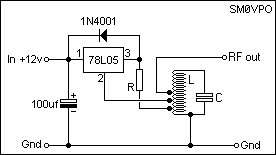Regulator used as an oscillator

The tuned circuit, L and C, determine the frequency of operation, and the resistor R should be chosen to get the required RF output. R should be set to 50% of the current the regulator will handle, for example 50mA (100 Ohms), which will give you about 10mW (+10dBm). If you use a bypass transistors (or a high current regulator) then you can get a lot more power out. The output tapping from the oscillator can be taken high up the coil, as shown, for a high-impedance output. If you want to get a higher power output then take the output tapping lower down, perhaps the junction of the resistor R. The tappings of the coil are typically 10% for the regulator (pin-3) output and 15% to 20% for the control pin (pin 2). Use the 20% tapping for a 50 Ohm output. The L and C impedance should be about 300 Ohms or so.

If you use a power regulator then this circuit can give you several watts of RF power. Just be careful to use the minimum tapping that will give you reliable oscillation, or you can generate a lot of distortion. Incidentally, this oscillator does not need a regulated voltage supply; it has an in-built regulator :-)

If you are interested, I found this circuit by accident. I built a voltage regulator, but tried to feed a bit of PSU ripple from the input circuit to cancel any low-level ripple at the output - I wanted a VERY low ripple PSU. Instead I created an oscillator after accidentally feeding the feedback to the control pin instead of the input pin.## Conclusion

I hope that you have found a couple of stimulating ideas with this article and hope that you can perhaps try out a few inventive ideas for yourself. Most of all, have fun.Very best regards from Harry Lythall
SM0VPO, Märsta, Sweden (QRA = JO89WO)
EA/SM0VPO, Nerja, Spain (QRA = IM86BS)